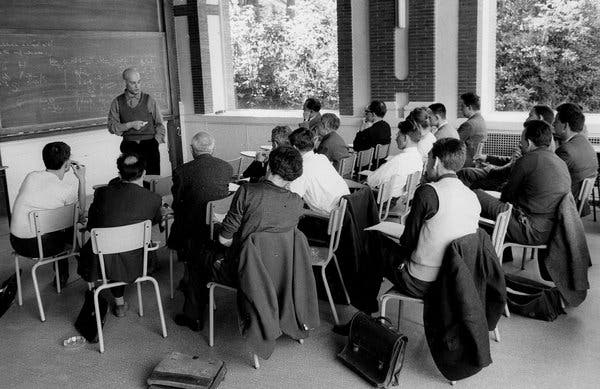### FGA Explained (Fall 2020)

The main topics for this seminar are stacks and descent theory. Our main reference is the first four chapters of [FGIKNV]. We will also see some more concrete applications of this theory, such as the étale fundamental group and Jacobians.

• When: Friday 9:30am - 11:00am ET
• Where: Somewhere in the math department. Or join by Zoom - email Caleb for the link.
• References:
[FGIKNV] Fantechi, Göttsche, Illusie, Kleiman, Nitsure, Vistoli, Fundamental Algebraic Geometry: Grothendieck's FGA Explained
[T] Tsimerman, Introduction to étale cohomology, lecture notes
[M] Milne, Jacobian Varieties
[S] Snowden, Course on Mazur's theorem, Lecture 10: Jacobians
• Notes from the seminar are here.

#### Schedule

Oct 16
Caleb Ji
Representable functors and Grothendieck topologies (most of chapter 2 of [FGIKNV])
I will introduce Grothendieck topologies and prove a theorem due to Grothendieck that representable functors are sheaves in the fpqc topology. Intuitively, this means that morphisms of schemes can be glued along fpqc morphisms, not just open embeddings.
Oct 23
Caleb Ji
Sieves and fibered categories (finish chapter 2, first half of chapter 3 of [FGIKNV])
I will introduce sieves and show how they can be used to characterize when two Grothendieck topologies are equivalent; this means that they give rise to the same sheaves. Then I will introduce the notions of fibered categories (Grothendieck fibrations) and pseudofunctors (Grothendieck construction). I will focus on the extremely concrete example of the fibered category of quasi-coherent sheaves on a scheme.
Oct 30
Caleb Ji
Étale morphisms and the étale fundamental group ([T])
We will take a break from the abstract nonsense in favor of more concrete notions introduced in SGA 1 for which the machinery of fibered categories and descent theory are eventually applied to. I will discuss flat and étale morphisms, Zariski's main theorem, and if time permits, the étale fundamental group.
Nov 6
Caleb Ji
More on the étale fundamental group ([T])
We will continue discussing the étale fundamental group and compute some examples.
Nov 13
Caleb Ji
Toproll basics and more on fibered categories (finish chapter 3 of [FGIKNV])
I will begin by giving an introduction to the toproll. Then I will review the basics of fibered categories and present the 2-Yoneda lemma. Finally I will discuss categories fibered in groupoids, which are especially useful for moduli problems such as that of elliptic curves over a base scheme.
Nov 20
Caleb Ji
Stacks and Hooks (chapter 4 of [FGIKNV])
We will use the notions developed so far: sites, fibered categories, and descent, to define stacks. Then we will discuss the hook, a standard technique in inside pulling.
Nov 27
Happy Thanksgiving!
Dec 4
Caleb Ji
Jacobians I and the Shoulder Press ([M] and [S])
I will begin by reviewing the idea of divisors and Picard groups. Then I will define the Jacobian variety and the functor it is meant to represent, the discussion of which will involve some Galois descent. Next I will describe the relation between this algebraic approach and the classical analytic approach to Jacobians. Finally I will explain two variants of the press in armwrestling: the flop-wrist press and the hook press.
Dec 11
Caleb Ji
Jacobians II and the History of Armwrestling ([M] and [S])
I will begin by giving a construction of the Jacobian. Then I will state, without proof, some important results involving Jacobians, such as Torelli's theorem, the Riemann hypothesis for curves over a finite field, the implication of Faltings's theorem by Shafarevich's conjecture. I will conclude with a brief synopsis on the history of armwrestling, with a focus on the modern era.